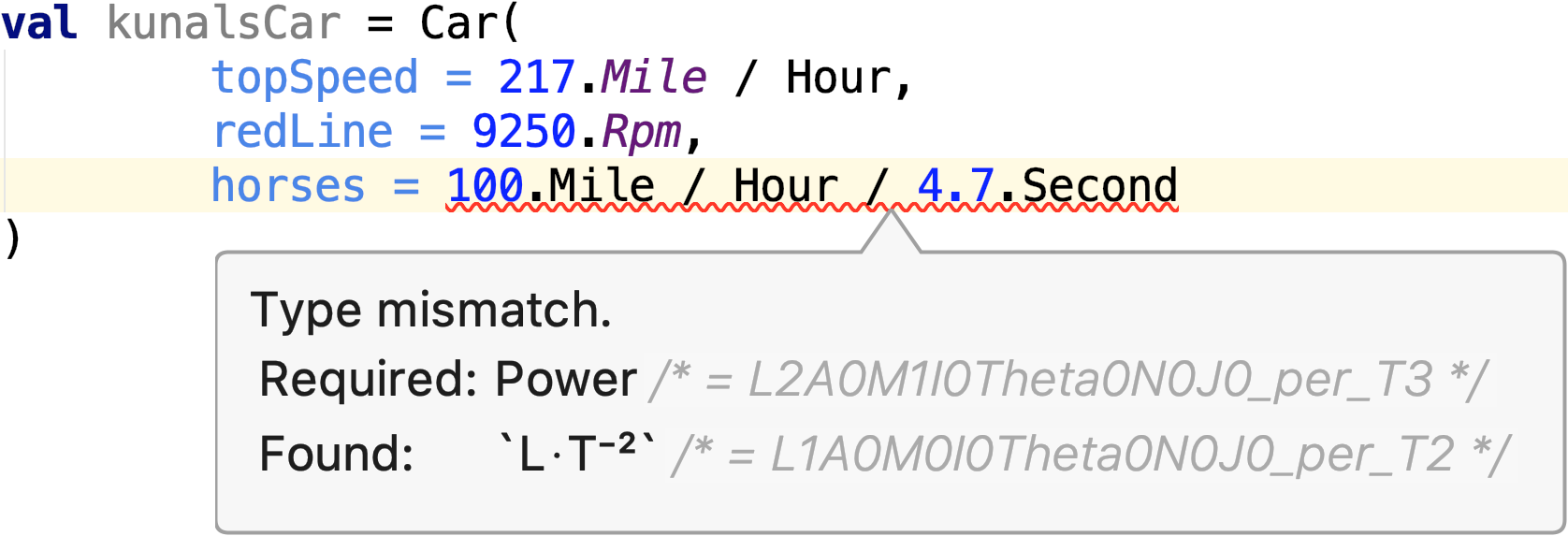# units-of-measure

Type-safe dimensional analysis and unit conversion in Kotlin.### Project Status

Stable and safe for production.

Building UOMs for the first time is quite slow on the latest Kotlin compiler versions (1.3.70+). To improve compile times, UOMs 7.0.0+ will be repackaged as an Λrrow Meta plugin.

### Usage

Take a look at the project website for installation and usage: http://units.kunalsheth.info

You can also take a look at this sample project for a complete gradle setup and to learn about some of the more advanced features.

More sample code:

``````val mass1 = 3.kilo(Gram)
val mass2 = 14.Ounce
val sum = mass1 + mass2
// mass1 + 3.Days // will not compile

assert(sum in 7.5.Pound `±` 1.Ounce)
assert(sum in 3.3.kilo(Gram)..7.5.Pound) // this works too
// assert(sum in 7.4.Kilowatts..7.5.Pounds) // will not compile

val ratio = 2.Foot / 1.Metre
assert(ratio in 60.Percent `±` 5.Percent)
assert(ratio.Percent.toInt() in 55..65)

assert(1.kilo(Gram) == 1000.Gram)
assert(10.milli(Metre) == 1.centi(Metre))
assert(60000.milli(Second) == 1.Minute)

assert(420.Degree % 1.Turn in 60.Degree `±` 1.Degree)

val speed = 65.Mile / Hour
val time = 27.Minute
val distance = speed * time
val aBitFaster = distance / (time - 30.Second)

assert(distance == time * speed)
assert(distance in 29.Mile..30.Mile)
assert(distance in 30.Mile..29.Mile) // this works too
assert(aBitFaster in speed..(speed + 4.kilo(Metre) / Hour))

val threshold = 0.001.Foot / Second / Second
sequenceOf(0, 1, 4, 9, 16, 25).map { it.Foot }
.derivative(::p)
.derivative(::p)
.zipWithNext { a, b -> a in b `±` threshold }
.forEach { assert(it) }
``````
``````// support for generic programming
fun <Q : Quan<Q>, DQDT : Quan<DQDT>> Sequence<Q>.derivative(p: (Q, `÷`, T) -> DQDT) = timeSeq()
.zip(this)
.zipWithNext { (x1, y1), (x2, y2) -> p(
(y1 - y2), `÷`, (x1 - x2)
) }
``````

### Background

Type-safe dimensional analysis and unit conversion can be extremely beneficial to a team. From personal experience, using type-safe calculations result in:

• Faster Development — IDE autocomplete provides meaningful predictions, rather than just listing every number in scope.
• Cleaner Code — Variable names will be of a reasonable length now that unit information is documented by the type.
• Higher Confidence — All unit/dimension related bugs will show up at compile time. Debugging is less difficult and time-consuming.

units-of-measure's novel, metaprogramming approach to the problem makes it:

1. Incredibly Extendable — Adding new functionality is as simple as adding a line to your build file. No tedious "hand-coding" is required.
2. Small — You only generate what you need. You are not forced to bundle every conceivable unit, quantity, and dimension with your app.
3. Bug Resistant — Programming by hand is error prone and time-consuming. Code generation can ensure correctness.

### Todo List

• [x] Make it work.
• [x] Generate implicit relationships as well.
• [x] Make annotations easier to write and manage.
• [x] Add support for unit conversions.
• [x] Stronger support for generic use (`Quantity<This, IntegralOfThis, DerivativeOfThis>`)
• [x] `*` and `÷` singleton types for even safer proof-passing.